Question

# Exercise 28.34 - Enhanced - with Feedback A closely wound, circular coil with radius 2.30 cm...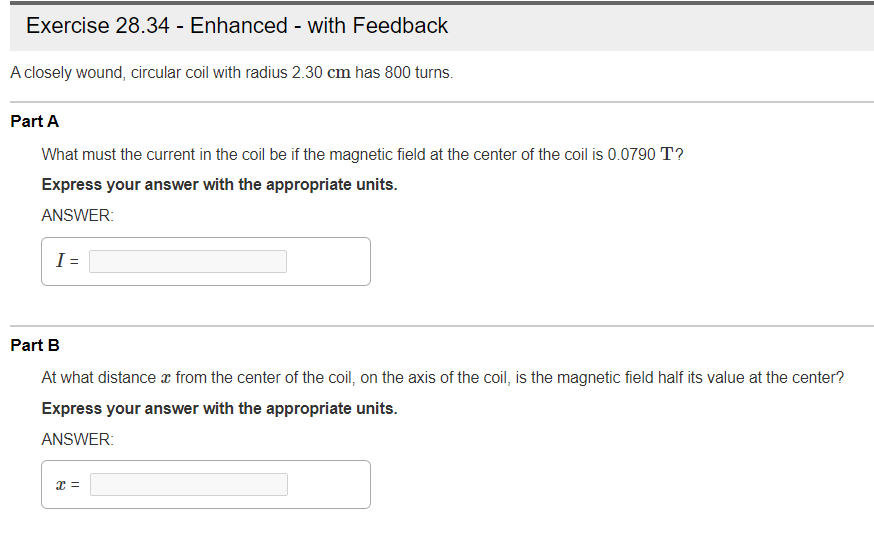Exercise 28.34 - Enhanced - with Feedback A closely wound, circular coil with radius 2.30 cm has 800 turns. Part A What must the current in the coil be if the magnetic field at the center of the coil is 0.0790 T? Express your answer with the appropriate units. ANSWER: I= Part B At what distance x from the center of the coil, on the axis of the coil, is the magnetic field half its value at the center? Express your answer with the appropriate units. ANSWER: 2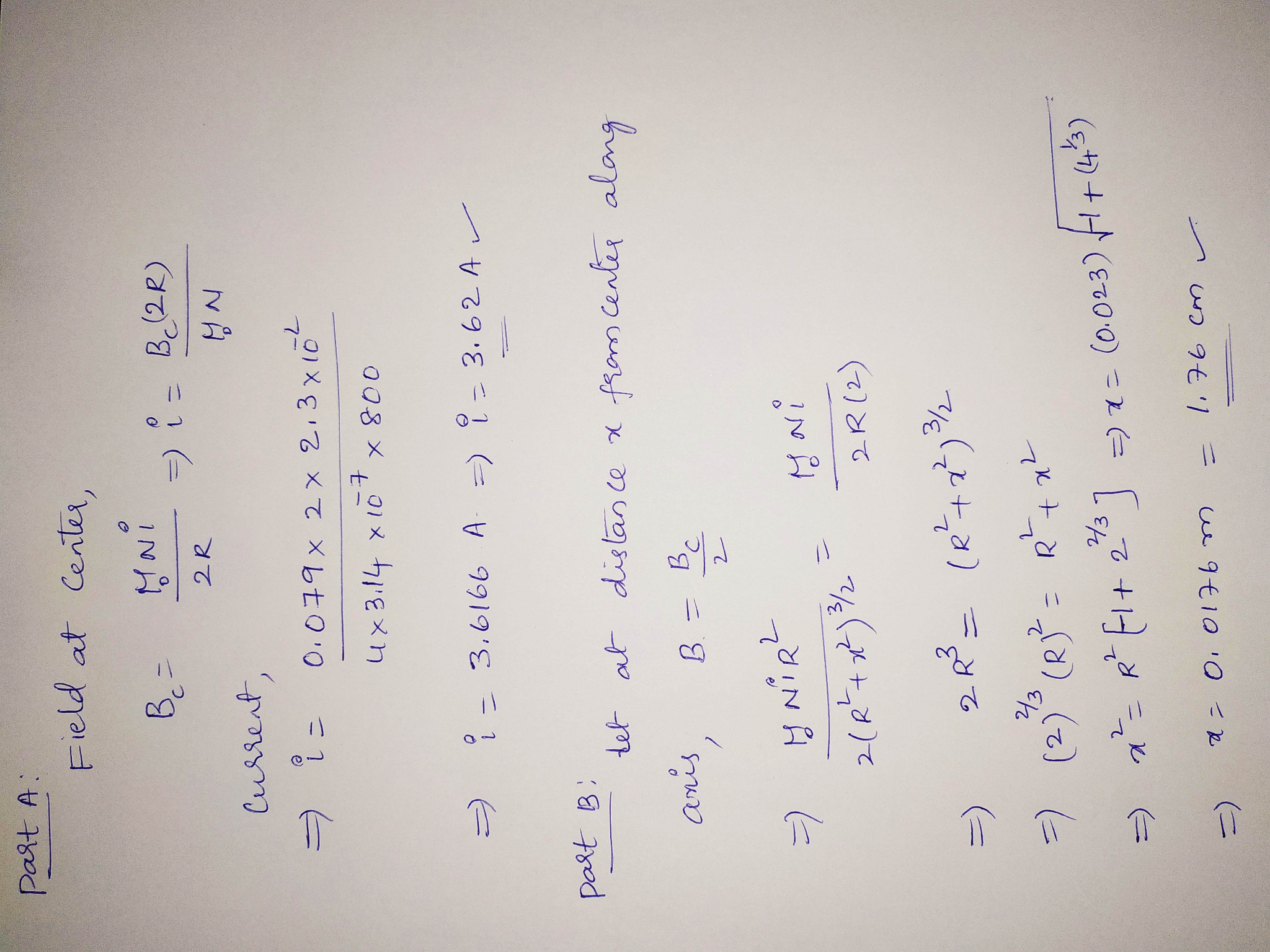#### Earn Coins

Coins can be redeemed for fabulous gifts.

Similar Homework Help Questions
• ### A closely wound, circular coil with radius 2.60 cm has 800 tums. Part A What must...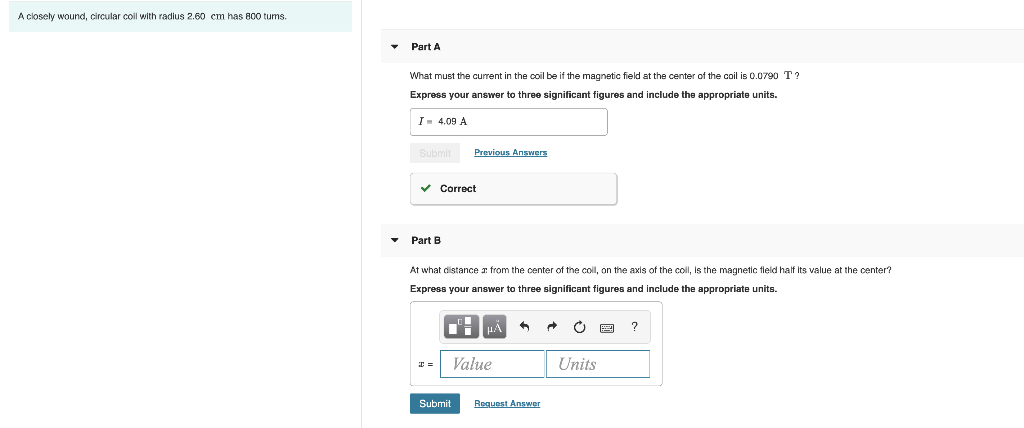A closely wound, circular coil with radius 2.60 cm has 800 tums. Part A What must the current in the coil be if the magnetic field at the center of the coil is 0.0790 T? Express your answer to three significant figures and include the appropriate units. I - 4.09 A Previous Answers ✓ Correct Part B At what distance from the center of the coil, on the axis of the coll, is the magnetic field half its value at...

• ### A closely wound, circular coil with radius 2.20 cm has 790 turns. Part A Part complete...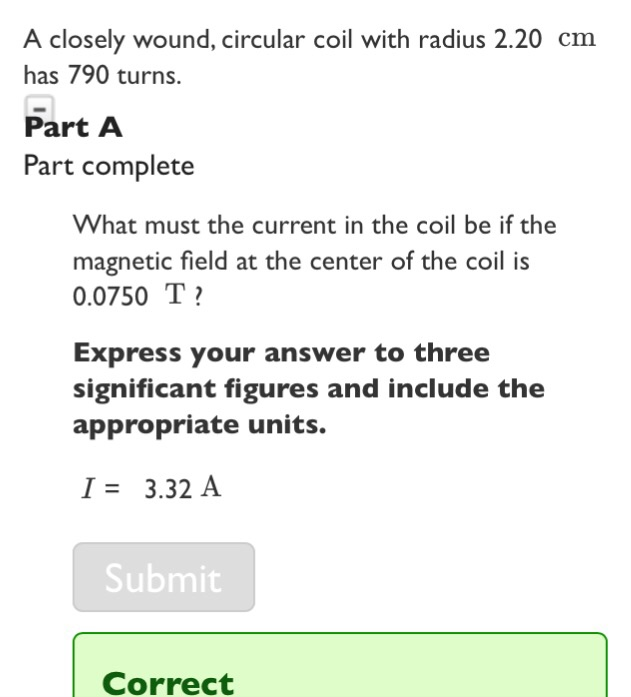A closely wound, circular coil with radius 2.20 cm has 790 turns. Part A Part complete What must the current in the coil be if the magnetic field at the center of the coil i:s 0.0750 T Express your answer to three significant figures and include the appropriate units. I3.32 A Submit Correct

• ### Exercise 28.36 21 of 25 Part A What must the current in the coil be if...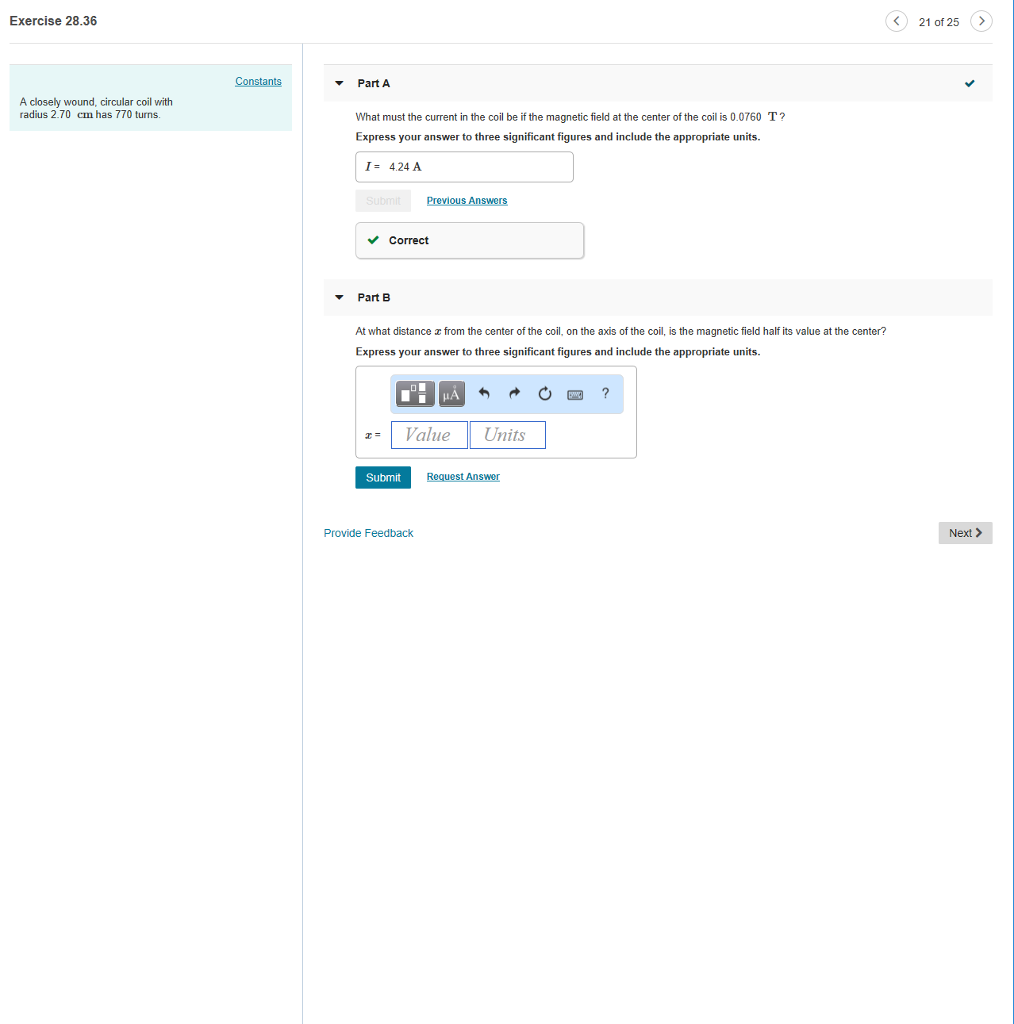Exercise 28.36 21 of 25 Part A What must the current in the coil be if the magnetic field at the center of the coil is 0.0760 T? Express your answer to three significant figures and include the appropriate units A closely wound, circular coil with radius 2.70 cm has 770 turns I-4.24 A Correct Part B At what distance x from the center of the coil, on the axis of the coil, is the magnetic field half its value...

• ### Reviel A closely wound, circular coil with a diameter of 4.00 cm has 690 turns and...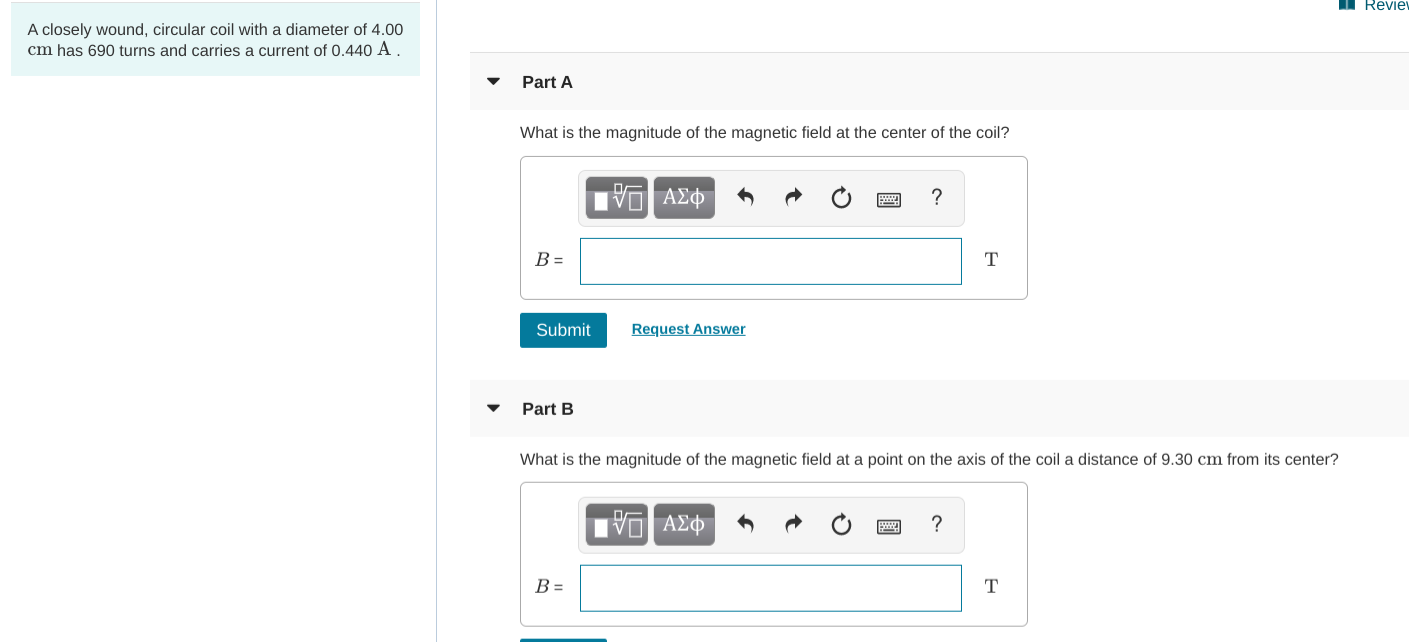Reviel A closely wound, circular coil with a diameter of 4.00 cm has 690 turns and carries a current of 0.440 A. Part A What is the magnitude of the magnetic field at the center of the coil? V AEO O ? B= Submit Request Answer Part B What is the magnitude of the magnetic field at a point on the axis of the coil a distance of 9.30 cm from its center? O AO O ?

• ### A closely wound circular coil with a diameter of 4.10 cm has 520 turns and carries...

A closely wound circular coil with a diameter of 4.10 cm has 520 turns and carries a current of 0.420 A. (a) What is the magnitude of the magnetic field at the center of the coil? T (b) What is the magnitude of the magnetic field at a point on the axis of the coil 8.00 cm from its center? T

• ### A closely wound circular coil with a diameter of 4.00 cm has 600 turns and carries...

A closely wound circular coil with a diameter of 4.00 cm has 600 turns and carries a current of 0.510 A . What is the magnetic field at the center of the coil?

• ### A closely wound circular coil with a diameter of 4.00cm has 600 turns and carries a current of...

A closely wound circular coil with a diameter of 4.00cm has 600 turns and carries a current of 0.500 A. What is the magnetic field at the center of the coil?

• ### A circular coil 13.0 cm in radius and composed of 145 tightly wound turns carries a...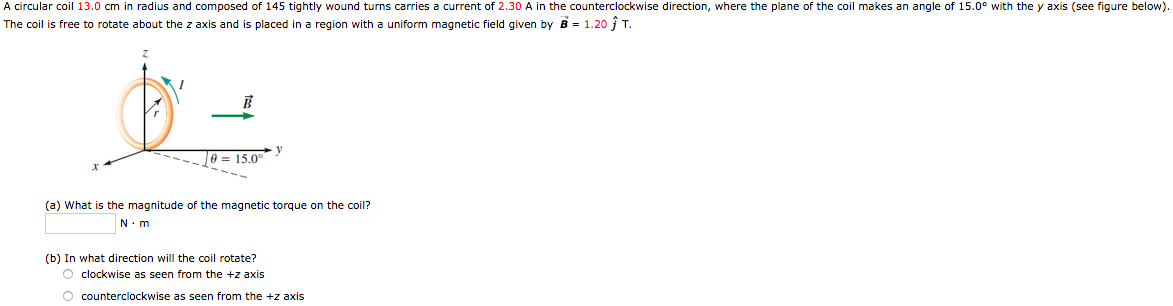A circular coil 13.0 cm in radius and composed of 145 tightly wound turns carries a current of 2.30 A in the counterclockwise direction, where the plane of the coil makes an angle of 15.0° with the y axis (see figure below). The coil is free to rotate about the z axis and is placed in a region with a uniform magnetic field given by B = 1.20 ſ T. Je = 15.0° (a) What is the magnitude of the...

• ### A 15cm long solenoid with radius 2.5 cm is closely wound with 600 turns of wire....

A 15cm long solenoid with radius 2.5 cm is closely wound with 600 turns of wire. The current in the windings is 8 A. What is the magnitude of the magnetic field generated in the center of the coil? (in mT= milli-Tesla)

• ### A circular coil 14.0 cm in radius and composed of 145 tightly wound turns carries a...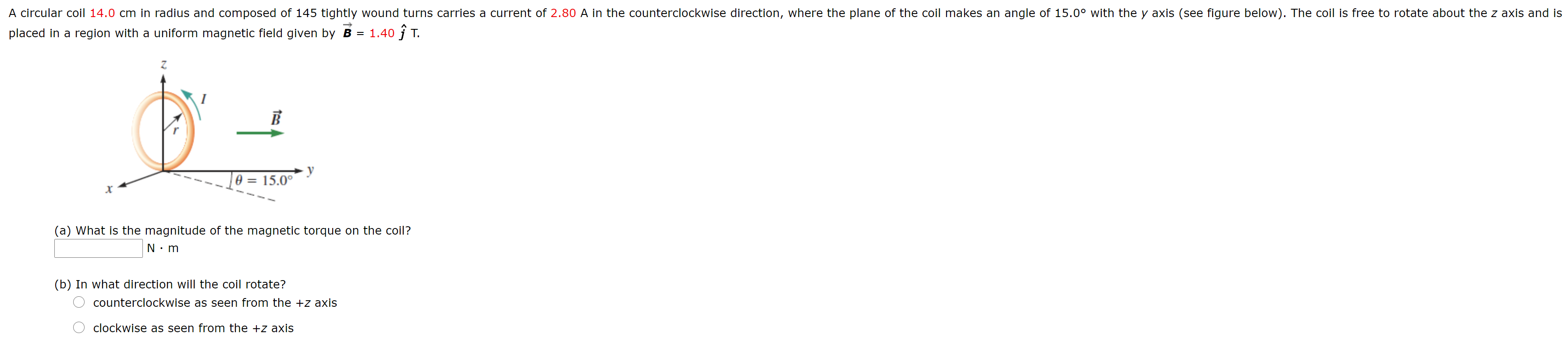A circular coil 14.0 cm in radius and composed of 145 tightly wound turns carries a current of 2.80 A in the counterclockwise direction, where the plane of the coil makes an angle of 15.0° with the y axis (see figure below). The coil is free to rotate about the z axis and is placed in a region with a uniform magnetic field given by B = 1.40 ſ T. B O = 15.0° (a) What is the magnitude of...